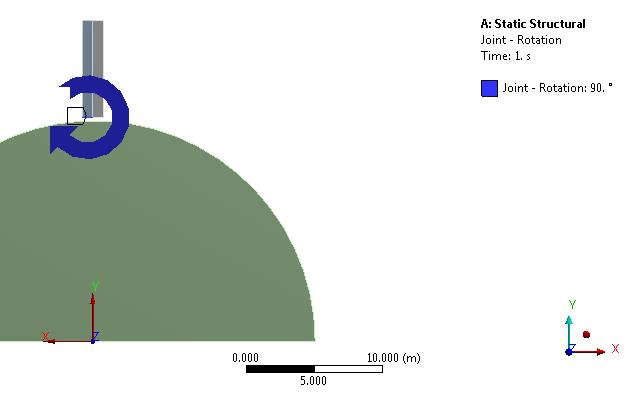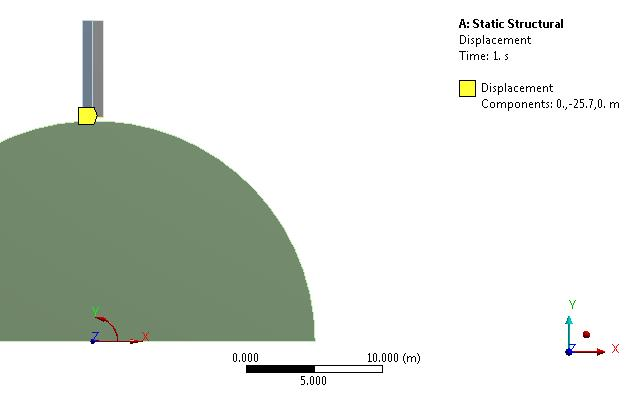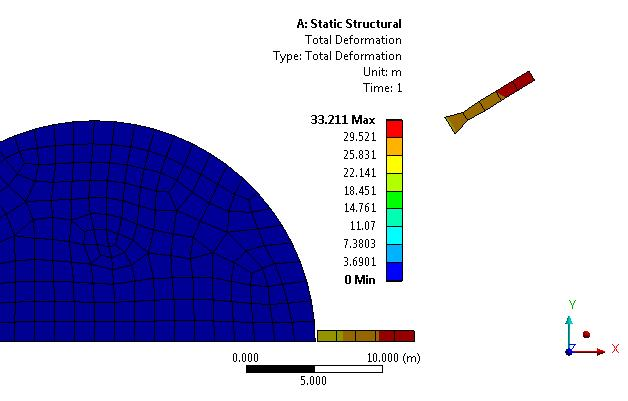peteroznewman
Subscriber

Hello Jon,

It's a good day when I find out I didn't really understand something ANSYS is doing. That happened today when I built a small model to test my assumption about how ANSYS does displacements in cylindrical coordinate systems.  I almost never use this capability, while I frequently use revolute joints, but I assumed they behaved basically the same. This small model shows they are not equivalent.

I built a model with two thin rectangles like the tips on the hands of a watch. The rectangle on the left has the bottom edge scoped to a revolute joint about the global coordinates at the center of the semicircle. The rectangle on the right has the bottom edge scoped to a displacement in the theta (Y) axis of the cylindrical coordinate system, while the radial (X) and axial (Z) displacements are set to zero.

The joint load is a 90 degree displacement.  The theta (Y) deformation on the bottom edge is -25.7, which is R*pi/2 since R = 16.4, and pi/2 is 90 degrees.The result surprised me.  I want to know how this unexpected result is computed.Perhaps someone who really understands this can explain it (or I could read the manual).

Regards,

Peter